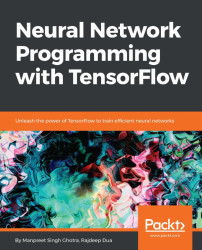•#### Neural Network Programming with Tensorflow#### Overview of this book

If you're aware of the buzz surrounding the terms such as "machine learning," "artificial intelligence," or "deep learning," you might know what neural networks are. Ever wondered how they help in solving complex computational problem efficiently, or how to train efficient neural networks? This book will teach you just that. You will start by getting a quick overview of the popular TensorFlow library and how it is used to train different neural networks. You will get a thorough understanding of the fundamentals and basic math for neural networks and why TensorFlow is a popular choice Then, you will proceed to implement a simple feed forward neural network. Next you will master optimization techniques and algorithms for neural networks using TensorFlow. Further, you will learn to implement some more complex types of neural networks such as convolutional neural networks, recurrent neural networks, and Deep Belief Networks. In the course of the book, you will be working on real-world datasets to get a hands-on understanding of neural network programming. You will also get to train generative models and will learn the applications of autoencoders. By the end of this book, you will have a fair understanding of how you can leverage the power of TensorFlow to train neural networks of varying complexities, without any hassle. While you are learning about various neural network implementations you will learn the underlying mathematics and linear algebra and how they map to the appropriate TensorFlow constructs.
Title PageCreditswww.PacktPub.comCustomer FeedbackPrefaceFree Chapter
Maths for Neural NetworksDeep Feedforward NetworksOptimization for Neural NetworksConvolutional Neural NetworksRecurrent Neural NetworksGenerative ModelsDeep Belief NetworkingAutoencodersResearch in Neural NetworksGetting started with TensorFlow## Introduction to RNNs

To understand RNNs, we have to understand the basics of feedforward neural networks. You can refer to Chapter 3, Optimization for Neural Networks, for details on feedforward networks. Both feedforward and recurrent neural networks are identified from the way they process the information or features through a series of mathematical operations performed at the various nodes of the network. One feeds information straight through (never touching a given node twice), the other cycles it through a loop.

A feedforward neural network is trained on image data until it minimizes the loss or error while predicting or classifying the categories for image types. With the trained set of hyper parameters or weights, the neural network can classify data it has never seen before. A trained feedforward neural network can be shown any random collection of images and the first image it classifies will not alter how it classifies the other images.

In a nutshell, these networks have no notion...